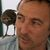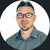# Calculate field type

2376
12
11-30-2017 03:43 PMbyEsri Contributor

Is there any information on the "calculate" field type? It is listed as a valid field type in the XLSForm "types" worksheet, but I can't find any information in the Survey123 help on this. And the "Calculations" sample uses the "calculation" column, but does not use the "calculate" field type.

Thanks!

Tags (2)
12 RepliesbyEsri Esteemed Contributor

Hi Shane,

As described in XLSForm essentials—Survey123 for ArcGIS | ArcGIS , calculate questions are designed to hold the results of calculations based on other questions.  For example, if you are inventorying vehicles, you can have separate questions for the number of passenger cars, trucks and cargo vans.  you can have a fourth question that is the total number of vehicles that adds together the previous 3.  Calculate questions are not displayed on the form, as they are automatically calculated without the survey taker's interaction.  More information on the type of calculations that can be done in a form is at Formulas—Survey123 for ArcGIS | ArcGIS .byEsri Contributor

Thanks, James!Occasional Contributor

Okay, can anyone tell me what the point of a "calculate" field type is?  You still have to add another field to feature layer if you want to use it.  What is the difference between that and just a hidden question?  What am i missing here?  They would actually be a really handy thing to have if you didnt have to add another field to your feature layer eveytime you wanted to use it. As far as i can tell though they are completely redundant.

ScottNew Contributor II

Hey Scott -

To avoid adding another field to your feature you can set the 'bindEsriFieldType' to null. This prevents the field from being a part of your feature. You can then use the calculation value from the calc field to populate a field in your form that DOES exist in the feature. It is a bit cumbersome and kind of roundabout. I typically like to perform calcs directly in the needed field; if that isn't practical then I default to javascript. Hope that is helpful!Occasional Contributor

Hi Chris.  Okay so if i set bindEsriFieldType to Null i an use the "calculate" field type as intended?  Awesome.  That's really handy. I knew there must be a logical reason for having it.  Thanks heaps!

ScottNew Contributor II

No problem! Glad I could help!New Contributor III

How do we take the result of the calculation and display as a field in the form?New Contributor II

Hey John - reference the value of the calc field by using its field name like so: \${myCalculatedField} in your formulas. So if you have a field called 'sum' that adds two other fields 'fieldOne' and 'fieldTwo', the calculation column for 'sum' would look like this: .=\${fieldOne} + \${fieldTwo}

Then to reference 'sum' somewhere else, you can use the field reference as the default value, like so: \${sum}

Does that help?New Contributor III

Hi Chris,

Im confused.  Here is how my excel is currently formatted.

type = calculation

name = surveylength

label = \${surveylength}

calculation field = \${surveylength} = \${endstation} - \${bgnstation}

begin station and end station are both type decimal and require user input.

what am I missing?